Courses

# Radioactivity (Part - 1) - Atomic and Nuclear Physics, Irodov JEE Notes | EduRev

## JEE : Radioactivity (Part - 1) - Atomic and Nuclear Physics, Irodov JEE Notes | EduRev

The document Radioactivity (Part - 1) - Atomic and Nuclear Physics, Irodov JEE Notes | EduRev is a part of the JEE Course I. E. Irodov Solutions for Physics Class 11 & Class 12.
All you need of JEE at this link: JEE

Q.214. Knowing the decay constant λ of a nucleus, find: (a) the probability of decay of the nucleus during the time from 0 to t; (b) the mean lifetime ζ of the nucleus.

Ans. (a) The probability of survival (i.e. not decaying) in time t is e-λt. Hence the probability of decay is 1 - e-λt

(b) The probability that the particle decays in time dt around time t is the difference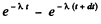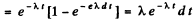Therefore the mean life time is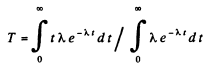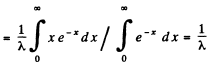Q.215. What fraction of the radioactive cobalt nuclei whose halflife is 71.3 days decays during a month?

Ans. We calculate λ first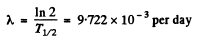Hence

fraction decaying in a month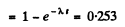Q.216. How many beta-particles are emitted during one hour by 1.0μg of Na24  radionuclide whose half-life is 15 hours?

Ans. Here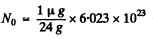= 2.51 x 1016

Also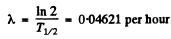So the number of β rays emitted in one hour is

No (1 - e-λt) = 1.13 x1015

Q.217. To investigate the beta-decay of Mg23  radionuclide, a counter was activated at the moment t = 0. It registered N1  beta-particles by a moment t1 = 2.0s, and by a moment t2  = 3t1 the number of registered beta-particles was 2.66 times greater. Find the mean lifetime of the given nuclei.

Ans.  If N0 is the number of radionuclei present initially, then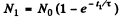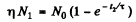where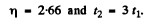Then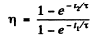or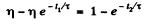Substituting the values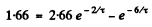Put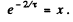Then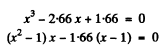or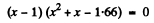Now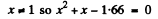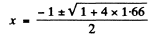Negative sign has to be rejected as x >0.

Thus   x = 0.882

This gives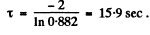Q.218. The activity of a certain preparation decreases 2.5 times after 7.0 days. Find its half-life.

Ans. If the half-life is T days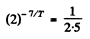Hence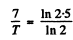or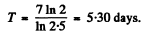Q.219. At the initial moment the activity of a certain radionuclide totalled 650 particles per minute. What will be the activity of the preparation after half its half-life period?

Ans.  The activity is proportional to the number of parent nuclei (assuming that the daughter is not radioactive). In half its half-life period, the number of parent nucli decreases by a factor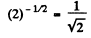So activity decreases to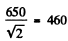articles per minute.

Q.220. Find the decay constant and the mean lifetime of Co55 radionuclide if its activity is known to decrease 4.0% per hour. The decay product is nonradioactive.

Ans.  If the decay constant (in (hour)-1 ) is λ., then the activity after one hour will decrease by a factor e Hence

0.96 = e

or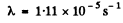= 0.0408 per hour

he mean life time is 24.5 hour

Q.221. A U238  preparation of mass 1.0 g emits 1.24.104 alphaparticles per second. Find the half-life of this nuclide and the activity of the preparation.

Ans.  Here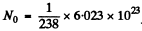= 2.531 X 1021

The activity is                  A = 1.24 x 104 dis/sec .

Then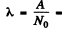4.90 x 1018 per sec .

Hence the half life is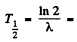4.49 x109 years

Q.222. Determine the age of ancient wooden items if it is known that the specific activity of C14 nuclide in them amounts to 3/5 of that in lately felled trees. The half-life of C14 nuclei is 5570 years.

Ans. in old wooden atoms the number of C14 nuclei steadily decreases because of radioactive decay. (In live trees biological processes keep replenishing C14 nuclei maintaining a balance. This balance starts getting disrupted as soon as the tree is felled.)

If T1/2 is the half life of C14 then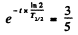Hence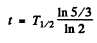= 4105 years ≈ 4.1x10years

Q.223. In a uranium ore the ratio of U238  nuclei to Pb206 nuclei is η = 2.8. Evaluate the age of the ore, assuming all the lead Pb206 to be a final decay product of the uranium series. The half-life of U238  nuclei is 4.5.10years.

Ans. What this implies is that in the time since the ore was formed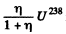nuclei have remained undecayed. Thus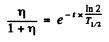or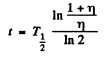Substituting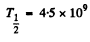years, η = 2.8

we get              t = 1.98 x 109 years.

Q.224. Calculate the specific activities of Na24  and U235  nuclides whose half-lifes are 15 hours and 7.1.10years respectively.

Ans. The specific activity of Na24 is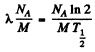= 3.22 x 1017 dis/(gm.sec)

Here M = molar w eight of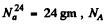is Avogadro num ber &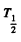is the half-life of  Na24

Sim ilarly the specific activity of U235 is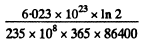= 0.793 x 10dis/(gm-s)

Q.225. A small amount of solution containing Na24  radionuclide with activity A = 2.0.103 disintegrations per second was injected in the bloodstream of a man. The activity of 1 cm3 of blood sample taken t = 5.0 hours later turned out to be A' = 16 disintegrations per minute per cm3. The half-life of the radionuclide is T = 15 hours. Find the volume of the man's blood.

Ans. Let V = volume of blood in the body of the human being. Then the total activity of the blood is A' V. Assuming all this activity is due to the injected Na24 and taking account of the decay of this radionuclide, we get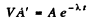Now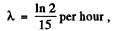t = 5 hour

Thus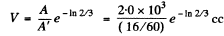= 5.99 litre

Q.226. The specific activity of a preparation consisting of radioactive Co58  and nonradioactive Co59 is equal to 2.2.1012 dis/(s•g). The half-life of Co58  is 71.3 days. Find the ratio of the mass of radioactive cobalt in that preparation to the total mass of the preparation (in per cent).

Ans. We see that

Specific activity of the sample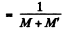{A ctivity of M gm of Co58 in the sample}

Here M and At are the masses of Co58 and Co59 in the sample. Now activity of M gm of Co58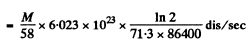= 1.168 x l015M

Thus from the problem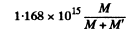= 2.2 x 1012

or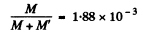Q.227. A certain preparation includes two beta-active components with different half-lifes. The measurements resulted in the following dependence of the natural logarithm of preparation activity on time t expressed in hours: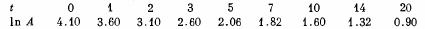Find the half-lifes of both components and the ratio of radioactive nuclei of these components at the moment t = 0.

Ans. Suppose N1 N2 are the initial number of component nuclei whose decay constants are λ1 , λ2 ( in (hour)-1 Then the activity at any instant is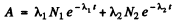The activity so defined is in units dis/hour. We assume that data In A given is of its natural logarithm. The daughter nuclei are assumed nonradioactive.
We see from the data that at large t the change in In A per hour of elapsed time is constant and equal to - 0.07. Thus

λ2 = 0.07 per hour

We can then see that the best fit to data is obtained by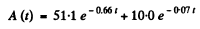[To get the fit we calculate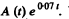We see that it reaches the constant value 10.0 at t = 7, 10, 14, 20 very nearly. This fixes the second term. The first term is then obtained by subtracting out the constant value 10.0 from each value of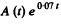in the data for small t ]

Thus we get  λ1 = 0.66 per hour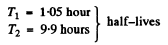Ratio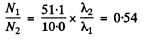Q.228. A P32  radionuclide with half-life T = 14.3 days is produced in a reactor at a constant rate q = 2.7.109 nuclei per second. How soon after the beginning of production of that radionuclide will its activity be equal to A = 1.0.109  dis/s?

Ans. Production of the nucleus is governed by the equation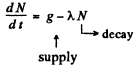We see that N will approach a constant value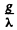This can also be proved directly. Multiply by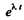and write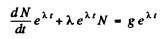Then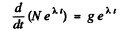or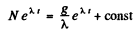At t = 0 when the production is starteed, N = 0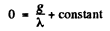Hence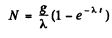Now the activity is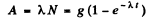From the problem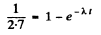This gives λt = 0.463

so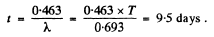Algebraically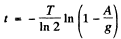Q.229. A radionuclide A1 with decay constant λ1 transforms into a radionuclide A2 with decay constant λ2. Assuming that at the initial moment the preparation contained only the radionuclide A1, find:
(a) the equation describing accumulation of the radionuclide A2 With time;
(b) the time interval after which the activity of radionuclide A2 reaches the maximum value.

Ans. (a) Suppose N1 and Nare the number of two radionuclides A1, A2 at time t. Then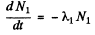(1)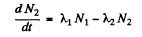(2)

From (1)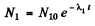where N10 is the initial number of nuclides Al at time t = 0

From (2)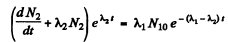or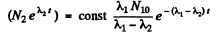since         N= 0 at t = 0

Constant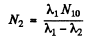Thus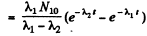(b) The activity of nuclide A2 is λ2 N2. This is maximum when N2 is maximum. That happens when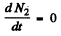This requires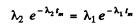or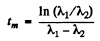Q.230. Solve the foregoing problem if λ1  = X= X.

Ans. a) This case can be obtained from the previous one on putting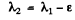where ε is very small and letting ε → 0 at the end. Then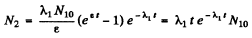or dropping the subscript 1 as the two values are equal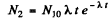(b) This is maximum when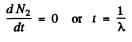Offer running on EduRev: Apply code STAYHOME200 to get INR 200 off on our premium plan EduRev Infinity!

111 docs

,

,

,

,

,

,

,

,

,

,

,

,

,

,

,

,

,

,

,

,

,

,

,

,

;